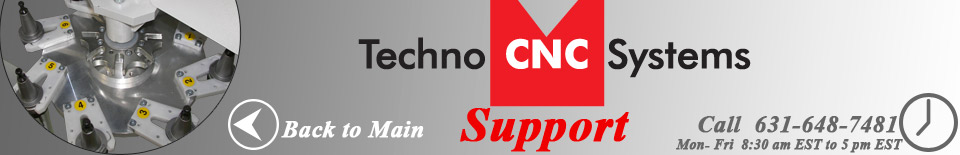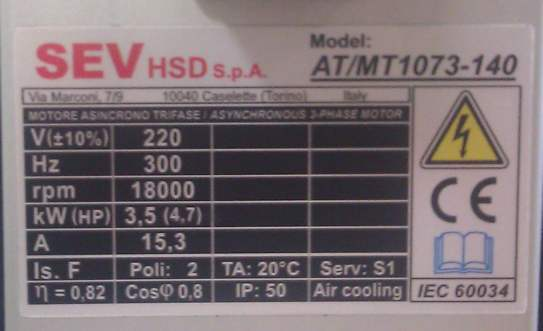On the front of all high frequency spindles (Columbo, HSD, etc) there is a nameplate that describes the attributes of the spindle (voltage, frequency, amperage, etc) What follows is an example of how to read the nameplate and obtain useful information. This nameplate:Shows the following useful information: (Note: the comma shown in these numbers should be thought of as a decimal point in the USA) V 220 means the spindle is 220 volts. NOTE: Other spindles may be 440 volts, and some are dual voltage (the voltage is selected by configuring the wiring inside the junction box). If the spindle is dual voltage, there will likely be two columns, dictating different characteristics (most notably amperage differences) for the different voltages. Hz 300 means the spindle max frequency is 300 Hz. rpm 18000 means this spindle max RPM is 18,000. kW (HP) means this spindle's wattage is 3500 watts, which is approximately 4.7 horsepower. A 15.3 means this spindle will draw no more than 15.3 amps during continuous use. The other symbols are typically not necessary for most users to know.